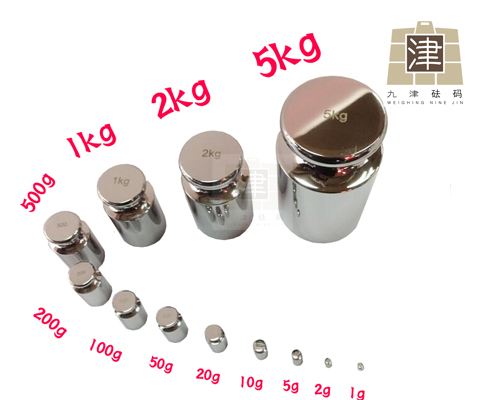## 新闻中心m ct = m cr + (V t - V r ) × ( ρ a - ρ o ) ± ΔI ×
m cs
ΔI S
± m cw

m cr ― ―标准砝码折算质量；
V t ― ―砝码的体积；
V r ― ―标准砝码的体积；
ρ a ― ―当地空气密度；
ρ o ― ―空气密度的参考值；
m cw ― ―添加小砝码的折算质量；
ΔI ― ―天平上读取的质量差值；
m cs ― ―检测天平灵敏度时放上的小砝码；
ΔI S ― ―灵敏度小砝码引起的天平示值。
3 标准不确定度分量的来源与评定
3.1 衡量过程的测量引起标准不确定度的评定
500g砝码进行10次测量，测量值见表1。
10 次测量的平均值为
- - - - -
Δm c =-0.90mg， 则 10 次测

u w (
- - - - -
Δm c ) =

i = 1
n
( Δm ci -
- - - - -
Δm c ) 2
n ( n - 1 )
=6.3×10 -2 mg

Δm ci ― ―第i次测量的质量差值；
- - - - -
Δm c ― ―多次测量的质量差值的平均值。
3.2 标准砝码引起的不确定度分量的评定
u r ( m cr ) = (
U
k
) 2 + u 2 inst ( m r )

500g砝码不确定度为U=0.24mg，k=2；
u inst ( m r ) ― ―标准砝码质量的不稳定性引

u inst ( m r ) 能够在标准砝码6次检测后，从质量变动中来

6次测量的平均值为 0.39mg， 由此不稳定性带来

u inst ( m r ) =

i = 1
n
( Δm ci -
- - - - -
Δm c ) 2
n ( n - 1 )
=0.027mg

1
-0.86
2
-1.03
3
-0.63
4
-0.63
5
-1.03
6
-1.23
7
-1.03
8
-0.83
9
-0.73
10
-1.03

1
0.3
2
0.5
3
0.4
4
0.4
5
0.37
6
0.37

50

2018年第 3期

u r ( m r ) = (
U
k
) 2 + u 2 inst ( m r ) =0.12mg
3.3 天平自身条件引起的不确定度的评定
3.3.1 灵敏度

u s = (
- - - - -
Δm c ) 2 (
u 2 ( m cs )
m 2 cs
+
u 2 ( ΔI S )
- - --
ΔI S
2
)
=3.9×10 -2 mg
- - - - -
Δm c ― ―被测砝码和标准砝码之间的平均折算

u ( m cs ) ― ―灵敏度砝码不确定度是其**大允许

u ( m cs ) =0.006/ （3×2）=0.001mg
m cs ― ―灵敏度砝码的折算质量值5.000g；
u ( ΔI S ) ― ― ΔI S 的标准不确定度，需对天平

4.4mg、4.6mg、4.4mg、5.1mg、4.4mg，用贝塞尔公式

u ( ΔI S ) =

i = 1
n
( ΔI si -
- - --
ΔI S ) 2
n ( n - 1 )
=0.14mg
- - --
ΔI S ― ―灵敏度砝码引起的衡量仪器指示值的改

3.3.2 衡量仪器显示的分辨力

u d = (
d/2
3
) × 2 =0.21mg
3.3.3 天平合成标准不确定度
u b = u s
2
+ u d
2 =0.21mg